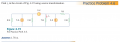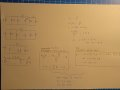# Source transformation principle - I am doing something wrong.

#### Xhendos

Joined Dec 1, 2018
9
Dear AAC,
I am trying to solve practive problem 4.6 which asks to find Io using source transformation principle as can be seen in the screenshot down below.What I tried to do was using source transformation principle to greatly simplify the circuit and then apply nodal analysis to get two constraint equations and find the voltage. Using that voltage V2 and subtract voltage 35/4 gets me the net voltage. I find the current flowing through that resistor 35/12 and calculate the amount of current flowing through the 7 ohm resistor since it is parallel with the 1 + 4 ohm resistors. My calculations can be seen below.However, when I solve I find that Io is equal to approximately 3.97A which does not match the book which says it is 1.78A. Could anyone point me on my mistake since I am stuck for quite a while and can't seem to find it.

#### RBR1317

Joined Nov 13, 2010
706
The only technique I ever use is nodal analysis, so this may be a dumb question, nevertheless if you are trying to find the current through the 7 ohm resistor, why do you transform away the 7 ohm resistor? How do you get the 7 ohm resistor back in order to find the current through it?

#### The Electrician

Joined Oct 9, 2007
2,914
You included too many resistors in your source transformations. Leave the 3 ohm and 7 ohm resistors alone. Convert the 5 A source which is in parallel with 6 ohms into a voltage source in series with 6 ohms. Convert the 3 A source which is in parallel with 5 ohms into a voltage source in series with 5 ohms. Now you can use mesh analysis to determine the 3 mesh currents, and from them you can get Io.

#### The Electrician

Joined Oct 9, 2007
2,914
Very good! Source transformations all the way. Now for more practice, solve it using both mesh analysis and nodal analysis.•Xhendos